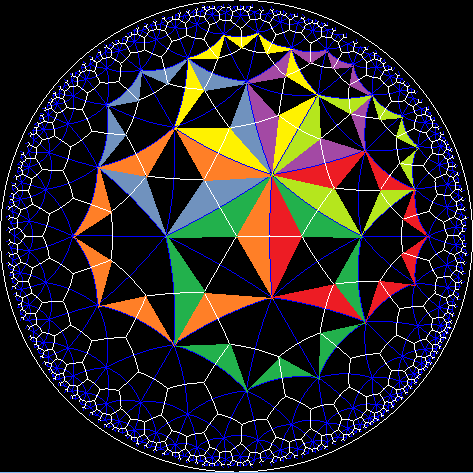Acronym ... Name hyperbolic order 7/2 heptagon tiling `` Circumradius sqrt[(1-cos2(π/7))/(3-4 cos2(π/7))] = 0.873057 i Vertex figure /2 Dual x7/2o7o Confer related tesselations: x3o7o

This hyperbolic star tiling can be obtained from x3o7o, when 7 triangles each are joined into one heptagon each. Every part of the tiling will be covered thrice: every triangle of that related tiling would be used in 3 heptagons each.

Incidence matrix according to Dynkin symbol

```o7/2o7x   (N → ∞)

.   . . | 2N |  7 |  7
--------+----+----+---
.   . x |  2 | 7N |  2
--------+----+----+---
.   o7x |  7 |  7 | 2N
```